# Branch Call Chapter 4 Sepehr Naimi www Nicer

• Slides: 47Branch & Call Chapter 4 Sepehr Naimi www. Nicer. Land. comTopics n n n Introduction to branch and call Branch Call n n n LR Calling a function Time Delay 2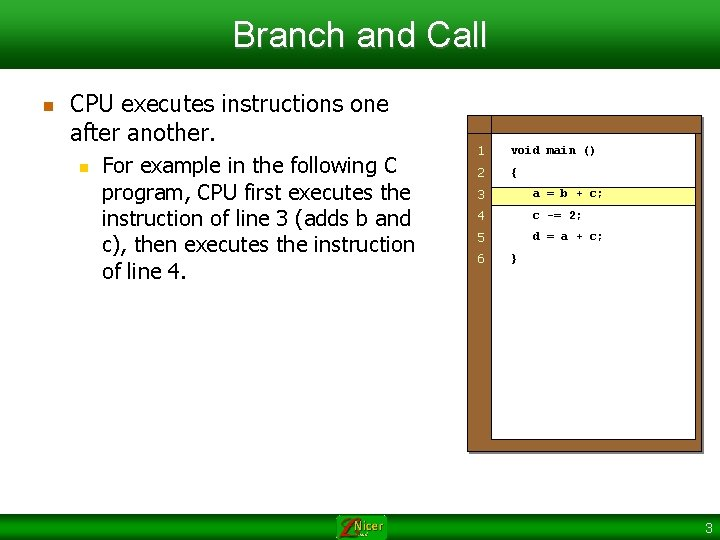Branch and Call n CPU executes instructions one after another. n For example in the following C program, CPU first executes the instruction of line 3 (adds b and c), then executes the instruction of line 4. 1 void main () 2 { 3 a = b + c; 4 c -= 2; 5 d = a + c; 6 } 3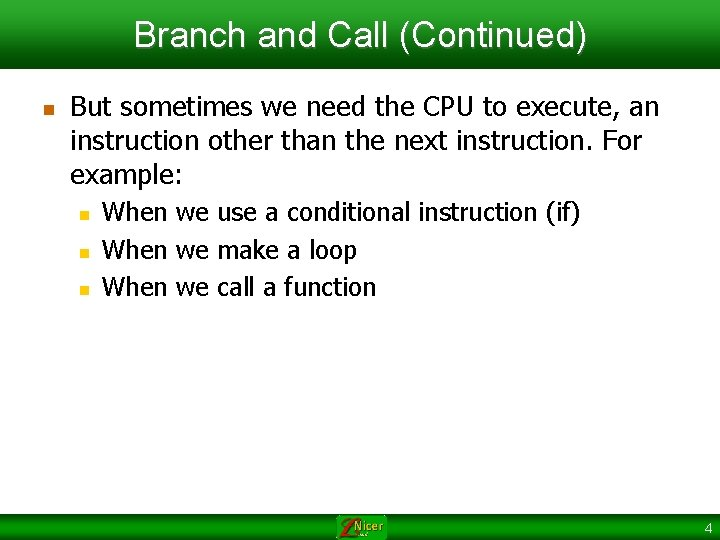Branch and Call (Continued) n But sometimes we need the CPU to execute, an instruction other than the next instruction. For example: n n n When we use a conditional instruction (if) When we make a loop When we call a function 4Branch and Call (Continued) n Example 1: Not executing the next instruction, because of condition. n In the following example, the instruction of line 6 is not executed. 1 void main () 2 { 3 int a = 2; 4 int c = 3; 5 if (a == 8) 6 c = 6; 7 else 8 c = 7; 9 c = a + 3; } 5Branch and Call (Continued) n Example 2: In this example the next instruction will not be executed because of loop. n In the following example, the order of execution is as follows: n n n Line 4 Line 5 Again, line 4 Again line 5 Line 6 1 void main () 2 { 3 int a, c = 0; 4 for(a = 2; a < 4; a++) 5 c += a; 6 7 a = c + 2; } 8 9 6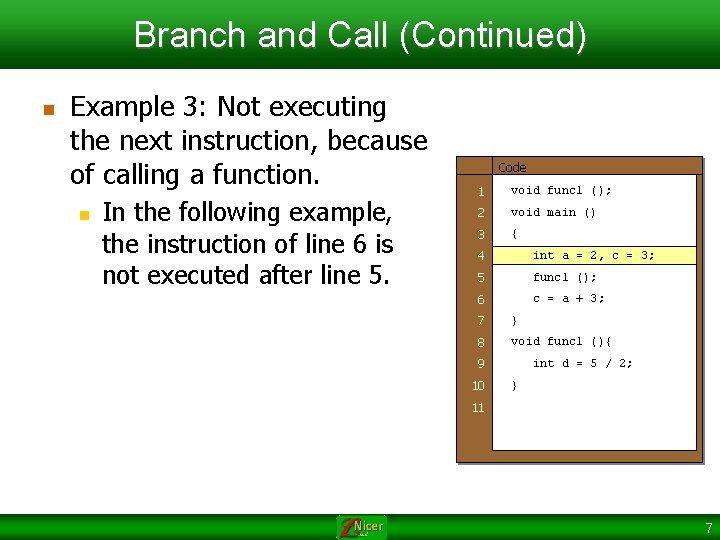Branch and Call (Continued) n Example 3: Not executing the next instruction, because of calling a function. n In the following example, the instruction of line 6 is not executed after line 5. Code 1 void func 1 (); 2 void main () 3 { 4 int a = 2, c = 3; 5 func 1 (); 6 c = a + 3; 7 } 8 void func 1 (){ 9 10 int d = 5 / 2; } 11 7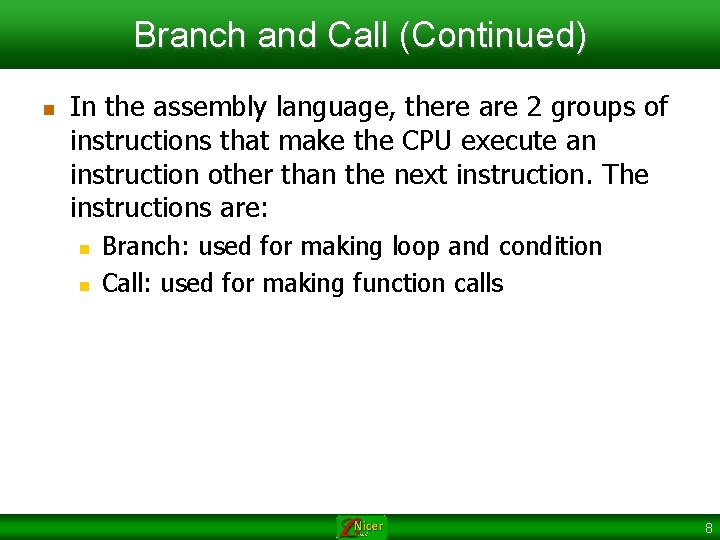Branch and Call (Continued) n In the assembly language, there are 2 groups of instructions that make the CPU execute an instruction other than the next instruction. The instructions are: n n Branch: used for making loop and condition Call: used for making function calls 8Branch n Branch changes the Program Counter (PC) and causes the CPU to execute an instruction other than the next instruction. 9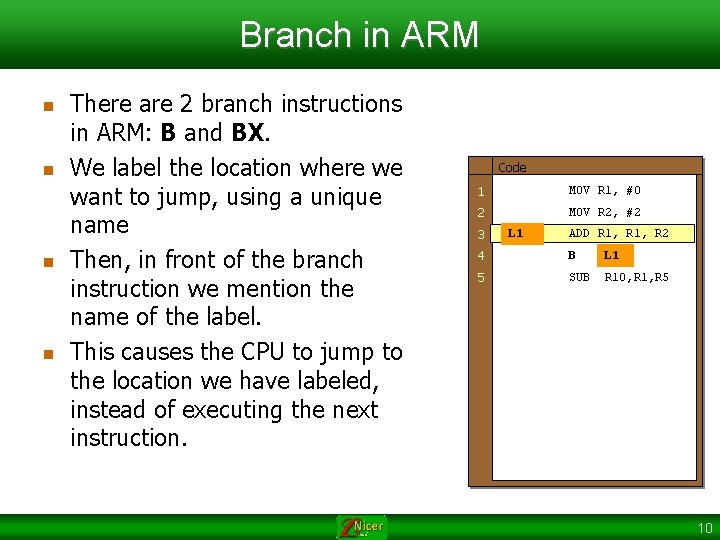Branch in ARM n n There are 2 branch instructions in ARM: B and BX. We label the location where we want to jump, using a unique name Then, in front of the branch instruction we mention the name of the label. This causes the CPU to jump to the location we have labeled, instead of executing the next instruction. Code 1 MOV R 1, #0 2 MOV R 2, #2 3 L 1 ADD R 1, R 2 4 B L 1 5 SUB R 10, R 1, R 5 10Ways of specifying the branch target n n B and BX provide the branch address using 2 different ways: B operand n n PC = PC + (operand*2) BX Rn n PC = Rn register 11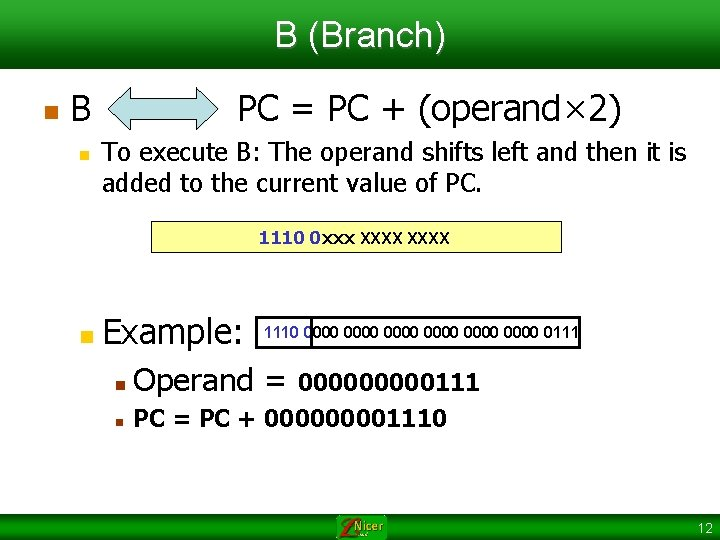B (Branch) n B n PC = PC + (operand× 2) To execute B: The operand shifts left and then it is added to the current value of PC. 1110 0 xxx XXXX n Example: 1110 0000 0000 0111 n Operand = n PC = PC + 00001110 00000111 12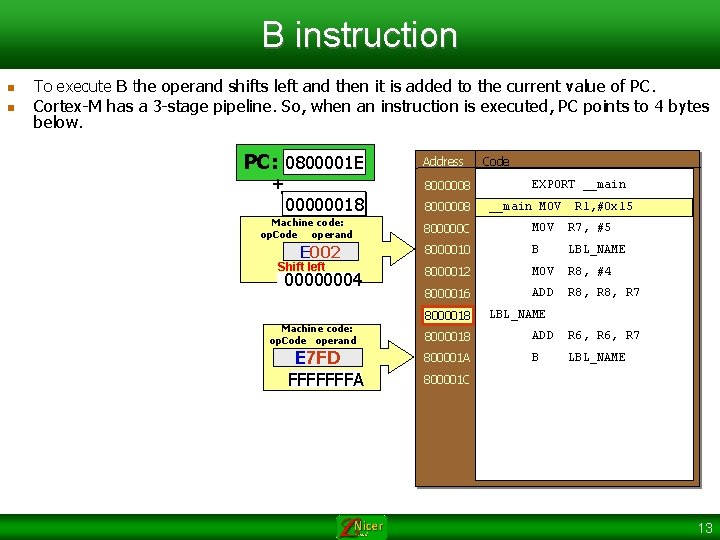B instruction n n To execute B the operand shifts left and then it is added to the current value of PC. Cortex-M has a 3 -stage pipeline. So, when an instruction is executed, PC points to 4 bytes below. 0800000 C 08000010 0800001 E 08000014 PC: 0800001 C + Address 8000008 00000018 Machine code: op. Code operand 8000008 Code EXPORT __main MOV R 1, #0 x 15 800000 C MOV R 7, #5 E 002 8000010 B LBL_NAME 00000004 8000012 MOV R 8, #4 8000016 ADD R 8, R 7 Shift left Machine code: op. Code operand E 7 FD FFFFFFFA 8000018 LBL_NAME 8000018 ADD R 6, R 7 800001 A B LBL_NAME 800001 C 13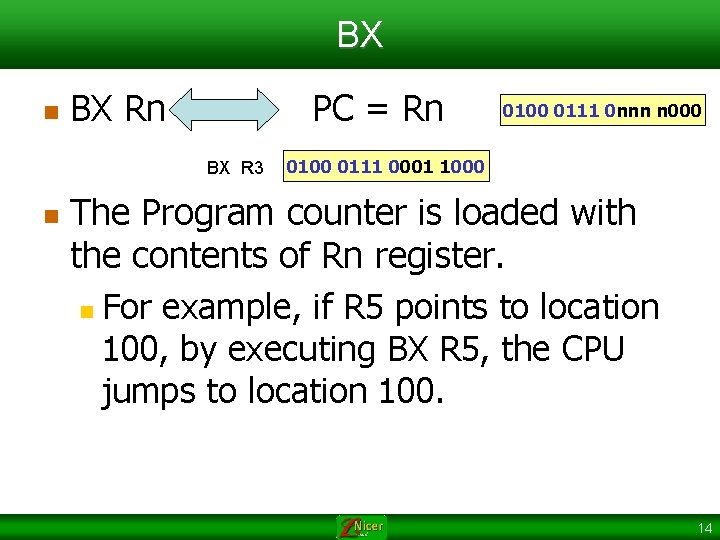BX n BX Rn PC = Rn BX R 3 n 0100 0111 0 nnn n 000 0111 0001 1000 The Program counter is loaded with the contents of Rn register. n For example, if R 5 points to location 100, by executing BX R 5, the CPU jumps to location 100. 14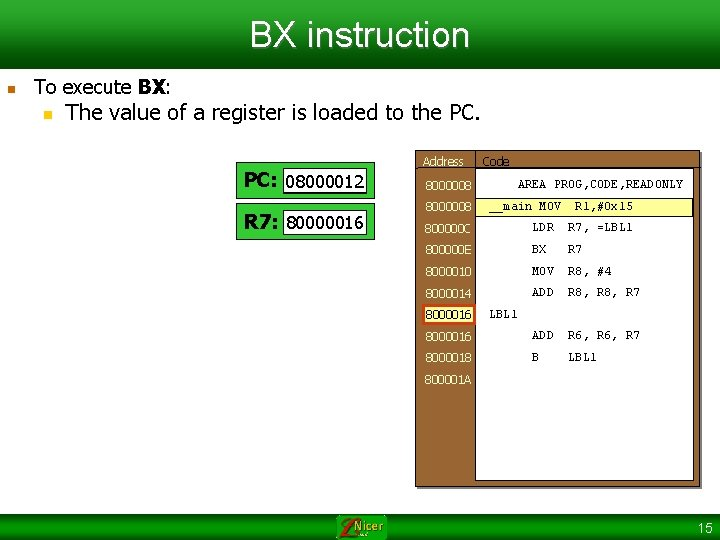BX instruction n To execute BX: n The value of a register is loaded to the PC. PC: 0800000 C 08000010 08000012 R 7: 0000 80000016 Address 8000008 Code AREA PROG, CODE, READONLY __main MOV R 1, #0 x 15 800000 C LDR R 7, =LBL 1 800000 E BX R 7 8000010 MOV R 8, #4 8000014 ADD R 8, R 7 8000016 ADD R 6, R 7 8000018 B LBL 1 8000016 LBL 1 800001 A 15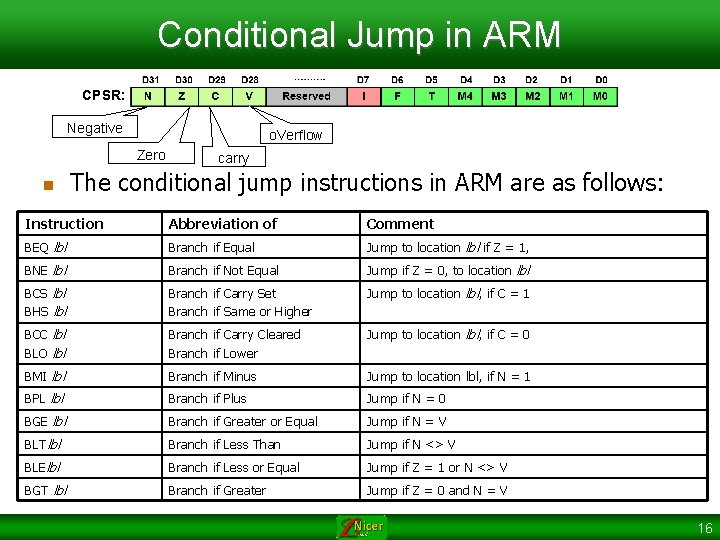Conditional Jump in ARM CPSR: Negative o. Verflow Zero n carry The conditional jump instructions in ARM are as follows: Instruction Abbreviation of Comment BEQ lbl Branch if Equal Jump to location lbl if Z = 1, BNE lbl Branch if Not Equal Jump if Z = 0, to location lbl BCS lbl BHS lbl Branch if Carry Set Branch if Same or Higher Jump to location lbl, if C = 1 BCC lbl BLO lbl Branch if Carry Cleared Branch if Lower Jump to location lbl, if C = 0 BMI lbl Branch if Minus Jump to location lbl, if N = 1 BPL lbl Branch if Plus Jump if N = 0 BGE lbl Branch if Greater or Equal Jump if N = V BLTlbl Branch if Less Than Jump if N <> V BLElbl Branch if Less or Equal Jump if Z = 1 or N <> V BGT lbl Branch if Greater Jump if Z = 0 and N = V 16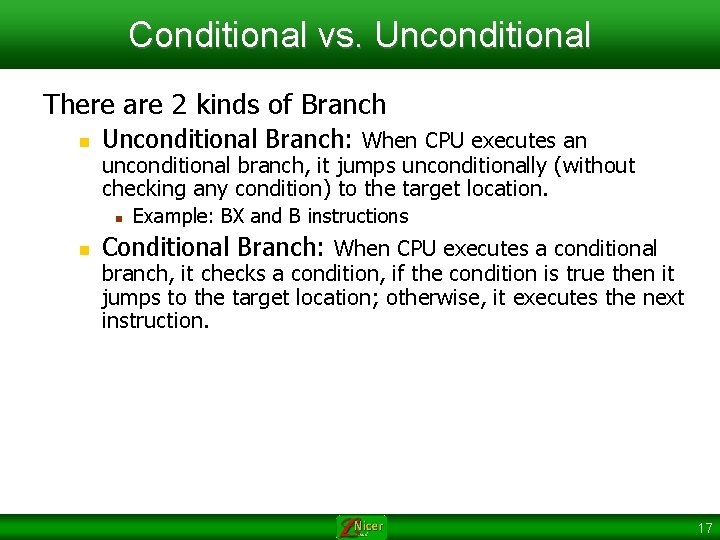Conditional vs. Unconditional There are 2 kinds of Branch n Unconditional Branch: When CPU executes an unconditional branch, it jumps unconditionally (without checking any condition) to the target location. n n Example: BX and B instructions Conditional Branch: When CPU executes a conditional branch, it checks a condition, if the condition is true then it jumps to the target location; otherwise, it executes the next instruction. 17Usages of Conditional jump n n Conditions Loop 18Conditions n When b is subtracted from a: n n The result is zero, when a is equal to b Carry will be set when a >= b a -b 19Example 1 n n Write a program that if R 0 is equal to R 1 then R 2 increases. Solution: SUBS R 0, R 1 BNE NEXT ADD R 2, #1 NEXT ; Z will be set if R 0 == R 1 ; if Not Equal jump to next 20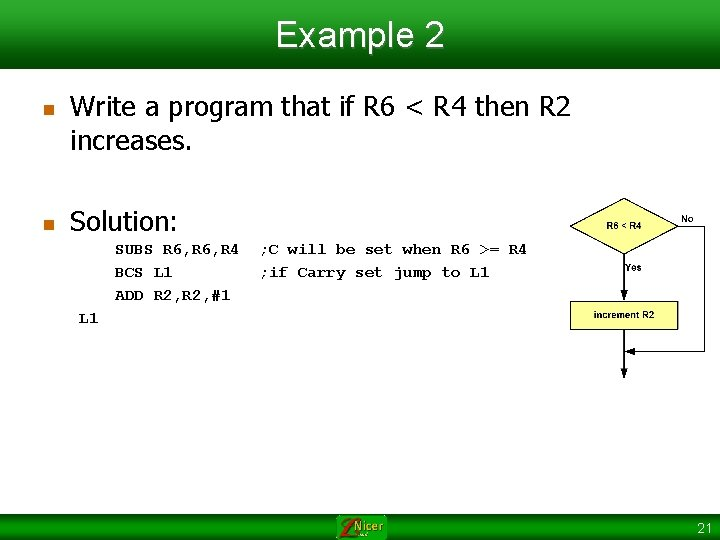Example 2 n n Write a program that if R 6 < R 4 then R 2 increases. Solution: SUBS R 6, R 4 BCS L 1 ADD R 2, #1 ; C will be set when R 6 >= R 4 ; if Carry set jump to L 1 21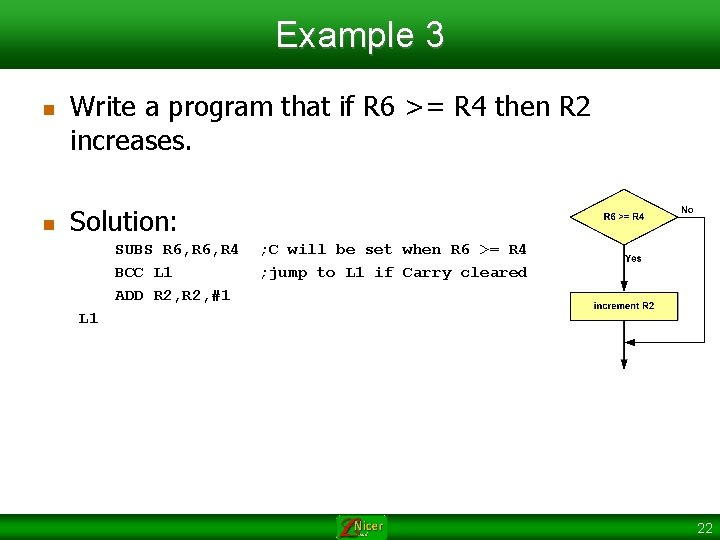Example 3 n n Write a program that if R 6 >= R 4 then R 2 increases. Solution: SUBS R 6, R 4 BCC L 1 ADD R 2, #1 ; C will be set when R 6 >= R 4 ; jump to L 1 if Carry cleared L 1 22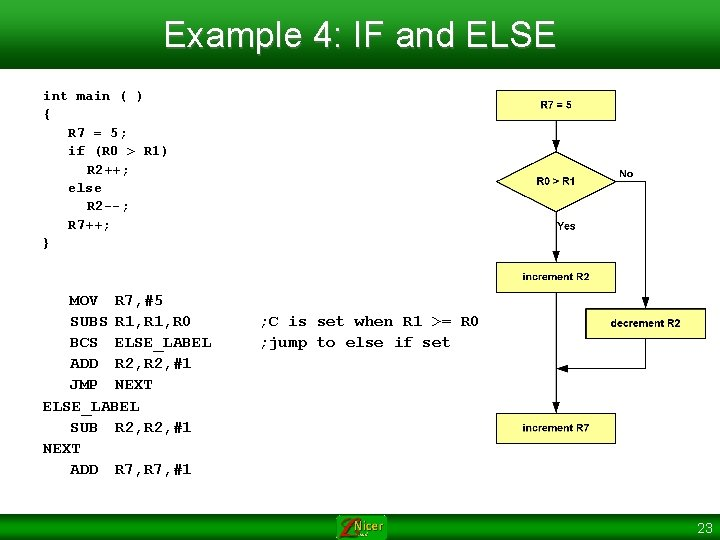Example 4: IF and ELSE int main ( ) { R 7 = 5; if (R 0 > R 1) R 2++; else R 2 --; R 7++; } MOV R 7, #5 SUBS R 1, R 0 BCS ELSE_LABEL ADD R 2, #1 JMP NEXT ELSE_LABEL SUB R 2, #1 NEXT ADD R 7, #1 ; C is set when R 1 >= R 0 ; jump to else if set 23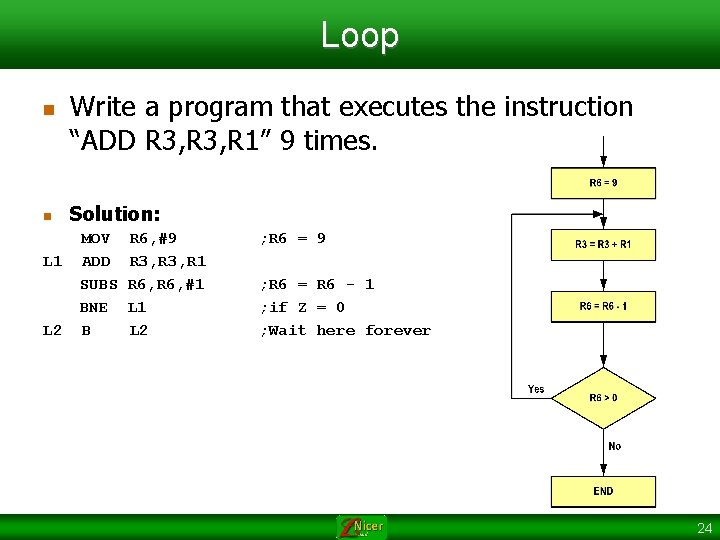Loop n n Write a program that executes the instruction “ADD R 3, R 1” 9 times. Solution: MOV R 6, #9 L 1 ADD R 3, R 1 SUBS R 6, #1 BNE L 1 L 2 B L 2 ; R 6 = 9 ; R 6 = R 6 - 1 ; if Z = 0 ; Wait here forever 24Loop n n Write a program that calculates the result of 9+8+7+…+1 Solution: MOV R 6, #9 MOV R 7, #0 L 1 ADD R 7, R 6 SUBS R 6, #1 BNE L 1 L 2 B L 2 @R 6 = 9 @R 7 = 0 @R 7 = R 7 + R 6 @R 6 = R 6 - 1 @if Z = 0 @Wait here forever 25Loop n n Write a program that calculates the result of 20+19+18+17+…+1 Solution: MOV R 6, #20 MOV R 7, #0 L 1 ADD R 7, R 6 SUBS R 6, #1 BNE L 1 L 2 B L 2 @R 6 = 20 @R 7 = R 7 + R 6 @R 6 = R 6 - 1 @if Z = 0 @Wait here forever 26Loop for (init; condition; calculation) { do something } init Do something calculation Yes Condition No END 27Loop n n Write a program that calculates 1+3+5+…+27 Solution: MOV R 0, #0 MOV R 6, #1 L 1 ADD R 0, R 6 ADD R 6, #2 ; R 16 = R 16 + 2 SUBS R 7, R 6, #27 BCC L 1 ; if R 6 <= 27 jump L 1 28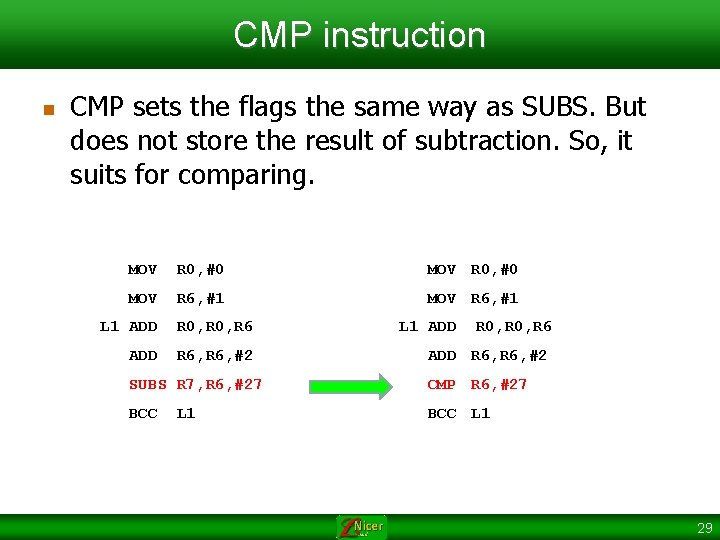CMP instruction n CMP sets the flags the same way as SUBS. But does not store the result of subtraction. So, it suits for comparing. MOV R 0, #0 MOV R 6, #1 L 1 ADD R 0, R 6 ADD R 6, #2 SUBS R 7, R 6, #27 CMP R 6, #27 BCC L 1 29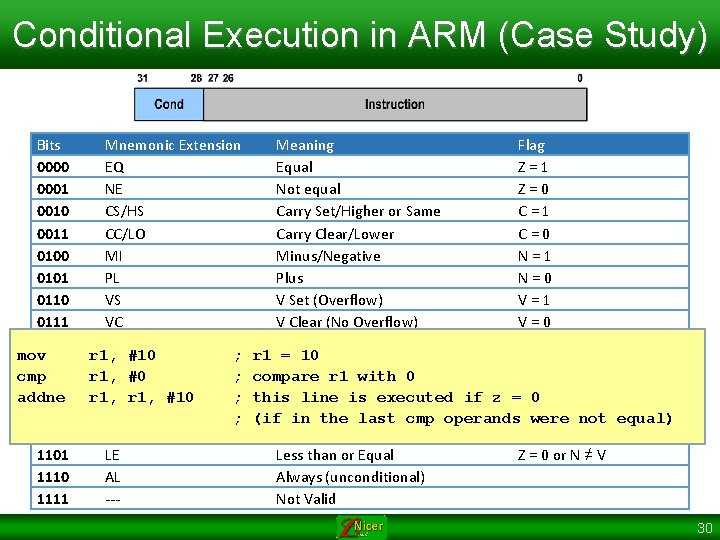Conditional Execution in ARM (Case Study) Bits 0000 0001 0010 0011 0100 0101 0110 0111 1000 mov 1001 cmp 1010 addne 1011 1100 1101 1110 1111 Mnemonic Extension Meaning Flag EQ Equal Z = 1 NE Not equal Z = 0 CS/HS Carry Set/Higher or Same C = 1 CC/LO Carry Clear/Lower C = 0 MI Minus/Negative N = 1 PL Plus N = 0 VS V Set (Overflow) V = 1 VC V Clear (No Overflow) V = 0 HI Higher C = 1 and Z = 0 r 1, #10 ; r 1 = 10 HS Lower or Same C = 1 and Z = 1 r 1, #0 ; compare r 1 with 0 GE Greater than or Equal N = V r 1, #10 ; this line is executed if z = 0 LT Less than N ≠ V ; (if in the last cmp operands were not equal) GT Greater than Z = 0 and N = V LE Less than or Equal Z = 0 or N ≠ V AL Always (unconditional) --Not Valid 30Calling a Function n To execute a call: n n Address of the next instruction is saved in LR (R 14). PC is loaded with the subroutine address Address Code 08000010 Machine code: F 000 F 803 op. Code Shiftoperand left 0006 AREA PROG, CODE, READONLY 08000010 __main MOV R 1, #5 08000014 MOV R 2, #6 08000018 BL FUNC_NAME 0800001 C MOV R 2, #6 08000020 L 1 B L 1 LR: 0800001 C 0000 08000022 FUNC_NAME 08000022 ADD R 2, R 1 PC: 0800001 C 08000014 08000028 08000024 0800002 C 08000018 08000024 SUB R 2, #3 08000028 BX LR + 0800002 A 08000022 31Time delay 32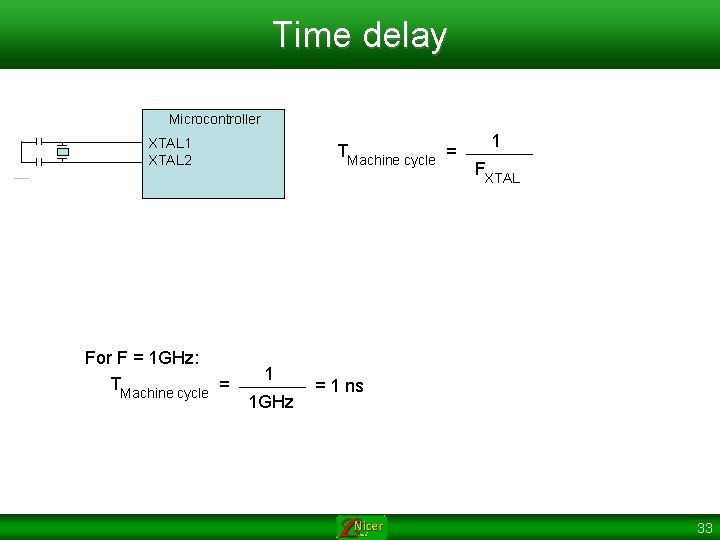Time delay Microcontroller XTAL 1 XTAL 2 For F = 1 GHz: TMachine cycle = 1 1 GHz 1 FXTAL = 1 ns 33Time delay machine cycle MOV R 6, #19 MOV R 0, #95 MOV R 1, #5 ADD R 6, R 0 ADD R 6, R 1 Delay = 5 x T machine cycle 1 1 1 5 = 5 x 1 ns = 5 ns 34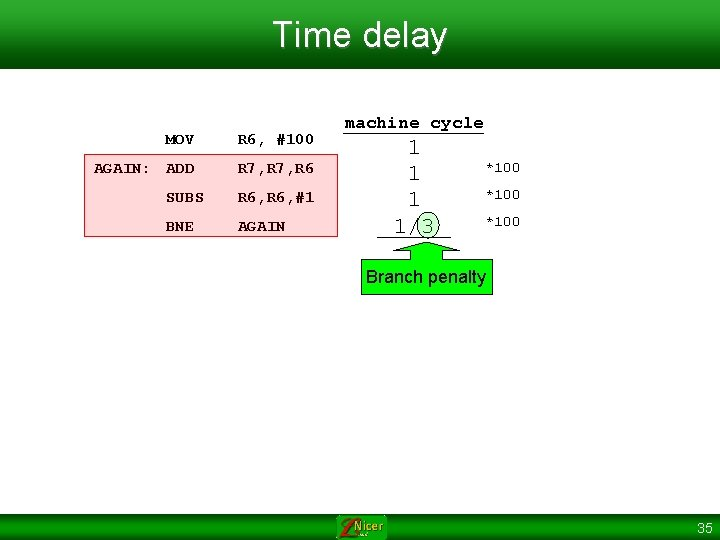Time delay MOV R 6, #100 AGAIN: ADD R 7, R 6 SUBS R 6, #1 BNE AGAIN machine cycle 1 1/3 *100 Branch penalty 35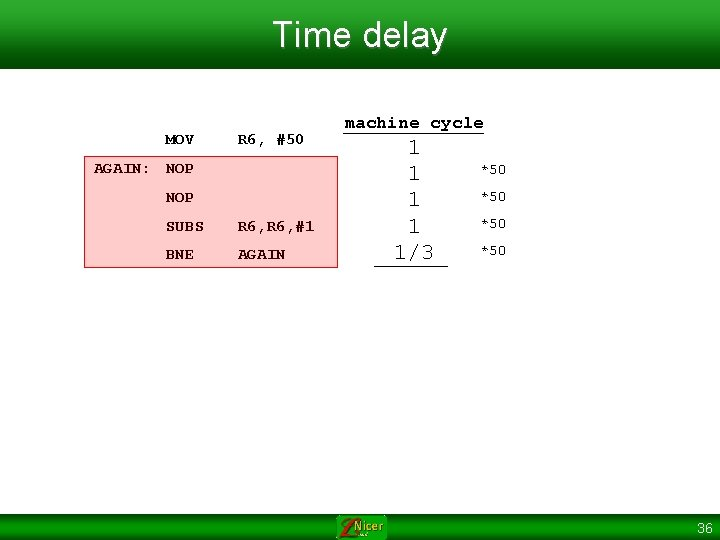Time delay MOV R 6, #50 AGAIN: NOP SUBS R 6, #1 BNE AGAIN machine cycle 1 1 1/3 *50 *50 36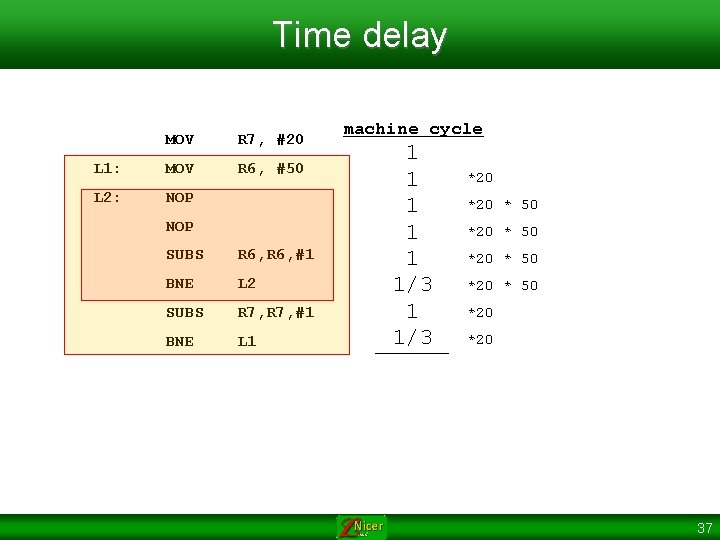Time delay MOV R 7, #20 L 1: MOV R 6, #50 L 2: NOP SUBS R 6, #1 BNE L 2 SUBS R 7, #1 BNE L 1 machine cycle 1 1 1/3 *20 * 50 *20 *20 37Stack 38Push and Pop Push Pop 39Stack Pointer n R 13 is the stack pointer n PUSH {Rn} n n SP = SP – 4 [SP] = Rn SP n 0 x 2000050 0 x 2000054 0 x 2000058 0 x 200005 C 0 x 2000060 0 x 2000064 POP {Rn} n n Rn = [SP] SP = SP + 4 40Push and Pop (Cont. ) PUSH {register list} n n n PUSH {R 10} PUSH {R 2, R 3, R 5} PUSH {R 5 -R 9} POP {register list} n n n POP {R 9} POP {R 4 -R 11} POP {R 1 -R 4, R 7} 41Stack Address Code. . . R 0: 00000010 R 1: 00000020 R 2: 00000030 0000 LDR R 0, =0 x 10 LDR R 1, =0 x 20 LDR R 2, =0 x 30 PUSH 0 x 00000010 {R 0} PUSH 0 x 00000020 {R 1} PUSH {R 2} 0 x 00000030 MOV R 0, #0 MOV R 1, #0 MOV R 2, #0 POP {R 2} SP 20005 F 0 20005 F 4 20005 F 8 20005 FC 2000600 POP {R 1} POP {R 0} L 1 B L 1 Memory 42Stack Initialization 43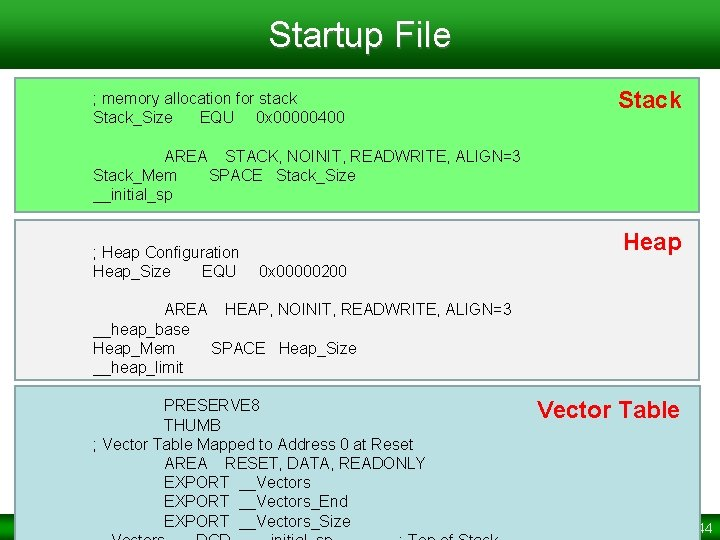Startup File ; memory allocation for stack Stack_Size EQU 0 x 00000400 Stack AREA STACK, NOINIT, READWRITE, ALIGN=3 Stack_Mem SPACE Stack_Size __initial_sp ; Heap Configuration Heap_Size EQU Heap 0 x 00000200 AREA HEAP, NOINIT, READWRITE, ALIGN=3 __heap_base Heap_Mem SPACE Heap_Size __heap_limit PRESERVE 8 THUMB ; Vector Table Mapped to Address 0 at Reset AREA RESET, DATA, READONLY EXPORT __Vectors_End EXPORT __Vectors_Size Vector Table 44Memory Allocation 45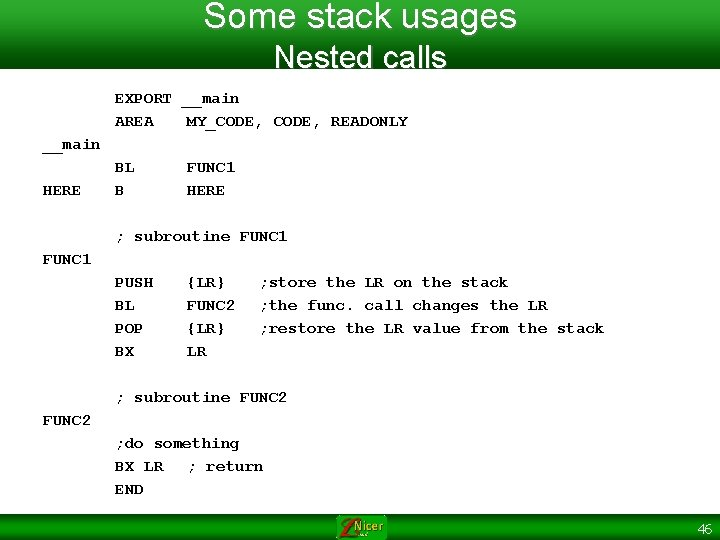Some stack usages Nested calls EXPORT __main AREA MY_CODE, READONLY __main HERE BL B FUNC 1 HERE ; subroutine FUNC 1 PUSH BL POP BX {LR} FUNC 2 {LR} LR ; store the LR on the stack ; the func. call changes the LR ; restore the LR value from the stack ; subroutine FUNC 2 ; do something BX LR ; return END 46Some stack usages Preserving registers values EXPORT __main AREA MY_CODE, READONLY __main HERE DELAY D_1 BL B DELAY HERE PUSH LDR SUBS BNE POP END {R 7} ; store R 7 onto the stack R 7, =120000 R 7, #1 D_1 {R 7} ; restore R 7 47